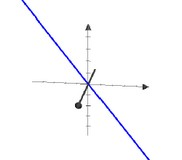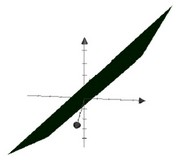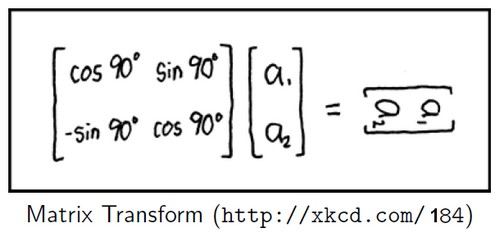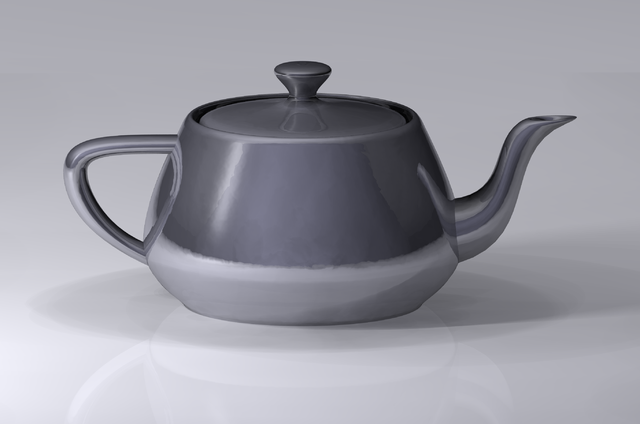# Geometry - Object

Viz - Graphic Object in Geometry

An object can be:

• a pre-defined shape
• or an arbitrary one defined with the help of a path.

## Notion

Notions such as:

• The length of a vector
• The angle between two vectors.

are introduced with the inner product of two vectors

## Object

### Containing the origin

A geometric object (point, line, plane, …) which contains the origin can be representing by a subset ofin two different ways :

• Span of some vectors
• Solution set of some system of linear equations with zero right-hand sides
Representation Line Plan
PictureSpan of some vectors
( subset of)
The Span of one “3-vectors” is
a line through the origin in three dimensions

Span {[1, 2, -2]}
The Span of two “3-vectors” is
a plane through the origin in three dimensions

Span {[4,-1,1], [0, 1, 1]}

This plane contains this two lines:
- Span {[4,-1,1]}
- Span {[0,1,1]}
Solution set of system
of linear equations
with zero right-hand sides
( subset of)
{[x, y, z] : [1,2,-2] . [x, y, z] = 0} {[x, y, z] : [4,-1, 1].[x, y, z] = 0, [0, 1, 1].[x, y, z] = 0}
• Point: Zero-dimensional: Span of the empty set: The origin

### Not containing the origin

A geometric object (point, line, plane, …) can be expressed as the solution set of a system of linear equations.

$\{x : \alpha_1 . x = \beta_1 , ... \alpha_n = \beta_n\}$

Discover MoreGeometry (Spatial, Linear Algebra)

A geometry is the geometric representation of an object in some coordinate system. A graphic is the combination of this objects in this coordinate system. Same as ! ...Geometry - Ellipse

An ellipse is a predefined shape. Under shear, a circle becomes an ellipse.Geometry - Node - Vertex (plural vertices)

Nodes of an object X,Y for two-dimensional objects Oracle Spatial: SDO_UTIL.GETVERTICES Table FunctionGeometry - Origin

All geometrics objects definition goes through the origin. In order to go through an another point, a translation (addition) must be performed.Geometry - Perpendicular (Right angle)

In elementary geometry, the word perpendicular describes the relationship between two geometric objects that meet at a right angle.Geometry - Rotation

Rotation is a geometric transformation and can be applied through the following transformation matrix Identity: Rotation matrixGeometry - Transformation

Transformation of geometric objects. A geometric transformation is represented by a transformation matrix. Transformations that keep the origin fixed are linear including: , ...Viz - Graphic Object

A graphic object is a geometrical object that: must be rendered. can get geometry transformation Graphical object can be grouped in order to maniuplate them as a unit. They can be place on a layer...